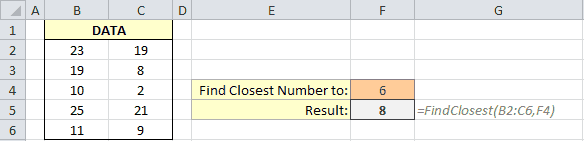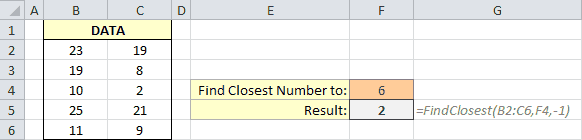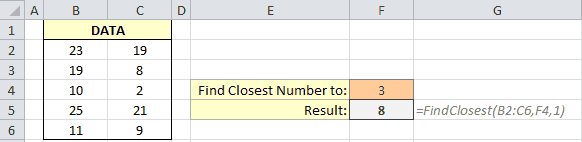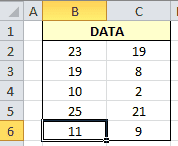Today I needed to find a value in a range of numbers that was closest to a certain target number. I needed to do this for hundreds of target numbers and a large 2D range. I’m sure some of you that are smarter at Excel than I am know how to do this without using VBA, but I don’t! Instead, I made this flexible UDF to do the work for me.

Pass this VBA function a range of cells and a target number that you want to find a number closest to and it will return the value in the range closest to your target. Not only that, the function includes a flag that lets you restrict your search to find the closest value below your target or the closest value above your target.

At the risk of sounding like a TV salesperson, here goes. “But wait! There’s more!” Because the output of the function is a variant, the address of the closest number can also be returned when calling this function from another VBA macro.

Play around with this custom Excel function. I think you’ll like it!

## Find the Closest Number in a Range

``````Function FindClosest(ByVal rng As Range, Target As Variant, Optional Direction As Integer) As Variant
'DEVELOPER: Ryan Wells (wellsr.com)
'DESCRIPTION: Function returns the nearest value to a target
'INPUT: Pass the function a range of cells, a target value that you want to find a number closest to
'       and an optional direction variable described below.
'OPTIONS: Set the optional variable Direction equal to 0 or blank to find the closest value
'         Set equal to -1 to find the closest value below your target
'         set equal to 1 to find the closest value above your target
'OUTPUT: The output is the number in the range closest to your target value.
'        Because the output is a variant, the address of the closest number can also be returned when
'        calling this function from another VBA macro.
t = 1.79769313486231E+308 'initialize
FindClosest = "No value found"
For Each r In rng
If IsNumeric(r) Then
u = Abs(r - Target)
If Direction > 0 And r >= Target Then
'only report if closer number is greater than the target
If u < t Then
t = u
Set FindClosest = r
End If
ElseIf Direction < 0 And r <= Target Then
'only report if closer number is less than the target
If u < t Then
t = u
Set FindClosest = r
End If
ElseIf Direction = 0 Then
If u < t Then
t = u
Set FindClosest = r
End If
End If
End If
Next
End Function``````

Make powerful macros with our free VBA Developer Kit

This is actually pretty neat. If you have trouble understanding or remembering it, our free VBA Developer Kit can help. It’s loaded with VBA shortcuts to help you make your own macros like this one - we’ll send a copy, along with our Big Book of Excel VBA Macros, to your email address below.

I'll take a free VBA Developer Kit

### How to use the FindClosest UDF

Copy and paste the above function into a module in your VBA editor to begin using the function. The function accepts 3 arguments, with the third argument being optional.

1. rng - The range of cells you want to search through to find the closest value.
2. Target - The target number that you want to find the value closest to. You can enter a number directly or refer to a cell.
3. Direction - An optional integer used to define how you want to search for the nearest value.
• Set Direction equal to 0 or blank to find the closest value
• Set Direction equal to -1 (or any negative number) to find the closest value less than or equal to your target
• Set Direction equal to 1 (or any positive number) to find the closest value greater than or equal to your target

#### Find Nearest Number

You’ll use this formula just like you would any other formula in Excel. In the following example, I have a 2D list of numbers and I want to find the number in the range closest to a 6. Cell F5 contains the formula:

``=FindClosest(B2:C6,F4)``

The result is an 8.Find Closest Number in Range

#### Find Nearest Smaller Number

What if I wanted to find the closest number less than or equal to 6? I would enter a formula like this into cell F5:

``=FindClosest(B2:C6,F4,-1)``

The negative number in the third argument instructs the function to only look for the nearest numbers below your target number. The result in this case is a 2.Find Closest Number Less Than or Equal To in Range

#### Find Nearest Larger Number

Entering a positive number in the Direction argument means the function will only look for the nearest number greater than or equal to your target:

``=FindClosest(B2:C6,F4,1)``

In this example I want to look for the closest number to a 3, but I only want to perform a one-sided search for numbers larger than 3.Find Closest Number Greater Than or Equal To in Range

This time, the result is an 8.

#### Typing Target Numbers Directly

One final thing before we move on. In all the prior examples, I pointed to a cell in my second argument. You don’t have to do this! You could type a number directly into the 2nd argument, like this:

``=FindClosest(B2:C6,2.7)``

#### Return Nearest Number

The same rules apply when you want to call this function from another VBA macro. We’ll use the same range of values we used during our Excel examples. Here’s how you would call the FindClosest function.

``````Sub Demo()
t = FindClosest(Range("B2:C6"), 14)
End Sub``````

A value of 11 will be stored in the variable t.

#### Return Address of Nearest Number

This is where it gets interesting. What if you don’t care what the nearest number is. Instead, you want to know where the nearest number is on your spreadsheet. This could be useful if you have thousands of data points in a 2D range of data since sorting would be impractical.

Because the FindClosest function returns a variant, when you call the function in VBA, you can invoke any of the properties and methods you can do with the `Range` object.

Take this macro for example:

``````Sub Demo2()
End Sub``````

Because we asked for the Address property, the result is the string \$B\$6 since that’s where the number 11 is stored.

#### Select Nearest Number

In this example, instead of returning the address, I want to select the cell containing the number closest to 14.

``````Sub Demo3()
FindClosest(Range("B2:C6"), 14).Select
End Sub``````VBA Select Nearest Number in Range

Sure enough. Cell B6 is selected!

You can really get creative here. I can imagine a scenario where it might be useful to highlight a cell that’s closest to a particular value. With my FindClosest function, you’ll be able to do just that!

## Closing Thoughts

I hope you enjoyed this VBA tutorial. I have more grab-and-go macro examples in my VBA Code Library so take a look!

That’s all for this tutorial. When you’re ready to take your VBA to the next level, subscribe using the form below.